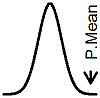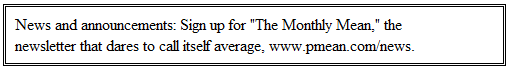P.Mean >> Category >>  Probability concepts (created 2007-09-21).These pages discuss some of the practical and theoretical considerations concerning probability. Also see Category: Bayesian statistics, Category: Statistical theory.

2016

12. P.Mean: The coin game (created 2016-10-15). The 538 website (http://fivethirtyeight.com) has a series of articles about election polling, sports predictions, and lots of other interesting things that are touched by Statistics. Once a week, they pose a puzzle, and this week's puzzle is quite intriguing.

2010

11. P.Mean: Sharing the same classroom for five consecutive years (created 2011-09-12). I needed to do a probability in in my head. I think I did it wrong and can't figure out. I'm trying to figure out what the probability of two kids out 50 that get divided into class each year. What is the chance that they would be in same class five years in a row. I thought it was 0.5 times 5. Or 2.5 But that was not right because 6 years in a row would be 3. What did I do wrong?

10. P.Mean: Another counter-intuitive probability problem (created 2010-07-04). A recent article in Science News, rekindled the two children problem and offered an odd twist. Here's the simple version. Suppose you have two children, one of whom is a boy. What is the probability that both children are boys? The obvious, but incorrect choice is 1/2. The correct answer is 1/3. How does this work?

2009

9. P.Mean: A sportswriter tackles the Monte Hall problem (created 2009-03-31). Joe Posnanski, a famous sports writer who loves statistics, wrote a couple of entries in his blog about the famous Monty Hall problem. I find the problem trite and annoying, but that probably says something more about me than about the problem. It is a very popular problem highly cited on the Internet and in many print publications. The Wikipedia quotes it from Parade magazine in 1990. Suppose you're on a game show, and you're given the choice of three doors: Behind one door is a car; behind the others, goats. You pick a door, say No. 1, and the host, who knows what's behind the doors, opens another door, say No. 3, which has a goat. He then says to you, "Do you want to pick door No. 2?" Is it to your advantage to switch your choice?

8. P.Mean: Interpreting a negative autocorrelation (created 2009-02-16). I have two questions regarding autocorrelation: if there is negative autocorrelation is it correct to say that "past values decreasingly influence future values? Why is positive auto-correlation considered more important by most statisticians.

2008

7. P.Mean: Interpretations for a two by two table (created 2008-10-18). I have a question. I have 2X2 table (below) and it shows a significant chi-square value for the overall test. I would now like to see where those differences occur using a method similar to a post-hoc analysis in ANOVA? Can this be done in SAS or other statistical software and, if so, how do I do it?

Outside resources:

1. Posnanski J. A Drunkard�s Walk Through Baseball Stats. Available at: http://joeposnanski.com/JoeBlog/2009/03/29/a-drunkards-walk-through-baseball-stats/  [Accessed March 31, 2009].
2. Posnanski J. A Brilliant Reader Question. Available at: http://joeposnanski.com/JoeBlog/2009/03/30/a-brilliant-reader-question/ [Accessed March 31, 2009].All of the material above this paragraph is licensed under a Creative Commons Attribution 3.0 United States License. This page was written by Steve Simon and was last modified on 2017-06-15. The material below this paragraph links to my old website, StATS. Although I wrote all of the material listed below, my ex-employer, Children's Mercy Hospital, has claimed copyright ownership of this material. The brief excerpts shown here are included under the fair use provisions of U.S. Copyright laws.

Definitions:

2007

6. Stats: Calculating probabilities involving correlated normal variables (June 4, 2007). Someone on EDSTAT-L asked about a problem involving differences of independent normal random variables. I am simplifying the problem a bit, but it essentially asked a question that was comparable to the following: Suppose you have three independent standard normal random variables: A, B, and C. What is the probability that A is smaller than B by one or more units and A is also smaller than B by one or more units.

5. Stats: Formulas for cumulative Poisson and binomial probabilities (February 19, 2007). I am updating some material about Poisson regression and noticed that some of the tests and confidence intervals rely on a percentile from a Chi-squared distribution or a gamma distribution. In previous work on binomial confidence intervals, I had noticed the use of a beta distribution and an F distribution. It seems odd to apply percentiles from continuous distributions for confidence intervals involving counting, but the formulas do indeed work. There are well known relationships for the cumulative distributions of the Poisson and binomial distributions that lead to these formulas.

2006

4. Stats: Extreme value distribution (January 9, 2006). I got an interesting question about an application in information theory of a statistical distribution called the Type I extreme value distribution. This distribution, also known as the Gumbel distribution, is useful for modeling the maximum or minimum of a large number of variables.

2005

3. Stats: Expected value and moments (July 29, 2005). Someone asked me what a statistical moment is. That's a rather technical term and is not needed except in rather theoretical and mathematical discussions. But it is still worth defining.

2. Stats: Geometric distribution (May 16, 2005). Someone asked me about a game where A, B, and C toss a coin in order until someone gets a heads on their coin flip. What are the probabilities that A will win? B will win? C will win?

2004

1. Stats: Testing multinomial proportions (November 9, 2004). I received an email inquiry about a problem that seems simple enough, but which just doesn't seem to have an easy answer. This person gave the following hypothetical data: Suppose in a sample of 100 people, 21 have blue eyes and 23 have green eyes. Can you test the hypothesis that the proportion of blue eyes is equal to the proportion of green eyes? This is not a two sample binomial problem and it is not a one sample binomial problem either. The only way you can properly analyze this data is to treat it as a single multinomial sample.

What now?

Browse other categories at this site